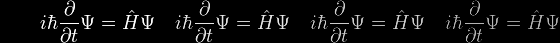# Eqn 11e Help

Given that ex/a = u + √(1+u2), we now invert both sides, which remain equal.

LHS: 1 / ex/a = e–x/a

 RHS: 1 / {u + √(1+u2)} = {u – √(1+u2)} / [{u – √(1+u2)} • {u + √(1+u2)}] = {u – √(1+u2)} / [u2 – (1+u2)] = {u – √(1+u2)} / [–1] = – u + √(1+u2) So e–x/a = – u + √(1+u2)

The technique used to clear the surdic denominator from the inverted RHS is frequently used in surdical operations, and is an ingenious variation on the difference between two squares: b2 – c = (b + √c) • (b – √c)